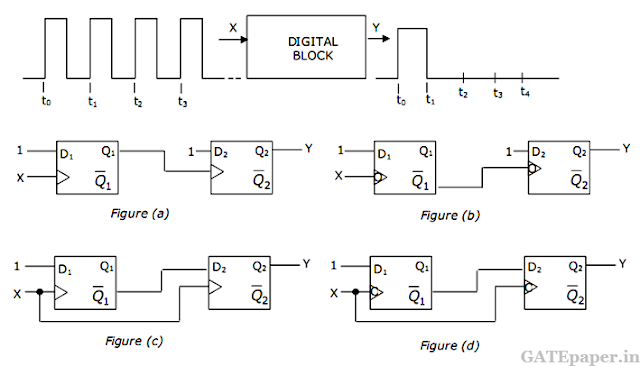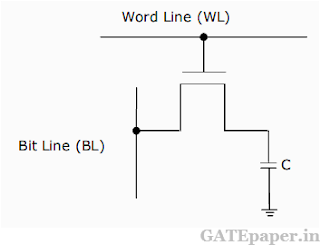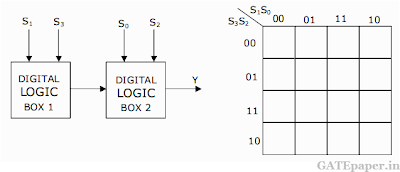### GATE 2001 ECE Video Solution on Digital Circuits (Digital Electronics)

1. The 2’s complement representation of – 17 is
a. 01110
b. 101111
c. 11110
d. 10001

2. For the ring oscillator shown in teh figure, the propagation delay of each inverter is 100 pico sec. What is the fundamental frequency of the oscillator output?a. 10 MHz
b. 100 MHz
c. 1 GHz
d. 2 GHz

3. An 8085 microprocessor based system uses a 4K x8 bit RAM whose starting address is AA00H. The address of the last byte in this RAM is
a. 0FFFH
b. 1000H
c. B9FFH
d. BA00H

4. In the figure, the LEDa. Emits light when both S1 and S2 are closed.
b. Emits light when both S1 and S2 are open.
c. Emits light when only of S1 or S2 is closed.
d. Does not emit light, irrespective of the switch positions.

5. In the TTL circuit in the figure, S2 to S0 are select lines and X7 to X0 are input lines. S0 and X0 are LSBs. The output Y is6. The digital block in the figure, realized using two positive edge triggered D flip-flops. Assume that for t<t0, Q1 = Q2 =0. The circuit in the digital block is given by7. In the DRAM cell in the figure is the VT of the N-MOSFET is 1 volt. For the following three combinations of WL and BL voltages.a. 5V; 3V; 7V
b. 4V; 3V; 4V
c. 5V; 5V; 5V
d. 4V; 4V; 4V

8. A monochrome video signal that ranges from 0 to 8 volts is digitized using an 8-bit ADC.
a. Determine the resolution of the ADC in V/bit.
b. Calculate the mean squared quantization error.
c. Suppose the ADC is counter controlled. The counter is up counter and positive edge triggered with clock frequency 1 MHz. What is the time taken in seconds to get a digital equivalent of 1.59 volts?

9. In the figure, the output of the oscillator, V1 has 10 volts peak amplitude with zero DC value. The transfer characteristic of the Schmitt inverter is also shown in the figure. Assume that the JK flip-flop is reset at time t=0.a. What is the period and duty cycle of the waveform V2?
b. What is the period and duty cycle of the waveform V3?
c. Sketch V1, V2 and V3 for the duration 0 ≤ t ≤ 6 µsec. Clearly indicate the exact timings when the waveforms V2 and V3 make high to low and low to high transitions.

10. For the digital block shown in the figure, the output Y = f(S3,S2,S2,S0), where S3 is MSB and S0 is LSB. Y is given in terms of minterms as Y = Σm(1,5,6,7,11,12,13,15) and its complement is given as Σm(0,2,3,4,8,9,10,14).a. Enter the logical values in the given Karnaugh map in the figure, for the output Y.
b. Write down the expression for Y in sum of products form using minimum number of terms.
c. Draw the circuit for the digital logic boxes using four 2 input NAND gates only for each of the boxes.

11. Consider the following sequence of instructions for an 8085 microprocessor based system.a. If the program execution begins at the location FF00H, write down the sequence of instructions which are actually executed till a HLT instruction. Assume all Flags are initially RESET.
b. Which of the three ports (PORT1, PORT2 and PORT3) will be loaded with data, and what is the bit pattern of the data?

Solution :